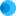Intuit HelpIntuit

# Common questions about the Form 1116 calculation in ProConnect

SOLVEDby IntuitUpdated June 12, 2023

## How is the pro rata share of home mortgage interest calculated?

Although ProConnect doesn't print copies of the worksheet, the program uses the Worksheet for Home Mortgage Interest - Line 4a found in the Form 1116 instructions to calculate the amount on this line.

To override the calculated value:

1. Go to Input Return Credits  Foreign Tax Credit (1116).
2. Scroll down to the Deductions and Losses section.
3. Enter the appropriate amount in Home mortgage interest [Override].

## Calculation of the Qualified Dividend Adjustment on Form 1116 Line 1a

In situations where the qualified dividends and/or capital gains are taxed in multiple tax brackets, the program calculates the adjustment for Form 1116, Foreign Tax Credit, Line 1a based on a ratio of rates between 5% and 15%. The ratio is calculated from the Qualified Dividends and Capital Gain Tax Worksheet or the Schedule D Tax Worksheet.

### How to calculate the total adjustment amount on Form 1116

1. Basically, the 5% ratio is the amount from the Qualified Dividends & Capital Gain Tax Worksheet, Line 10/Qualified Dividends & Capital Gain Tax Worksheet, Line 6.
2. The 15% ratio is 1 minus the just-calculated 5% ratio as shown in the preceding line.
3. The foreign capital gain distributions and the foreign qualified dividends are then multiplied by these respective ratios to come up with the amount that belongs to each rate.
4. The resulting amounts are then multiplied by 0.1429 for the 5% adjustment amount or 0.4286 for the 15% adjustment amount.
5. These results are then added together to come up with the total adjustment amount to report on Form 1116, Line 1a.
6. These percentages are per the Form 1116 instructions.

### Adjusting the foreign source qualified dividends or capital gain distributions taxed

• You adjust these amounts at the 0% rate by not including them on line 1a.
• Amounts taxed at the 0% rate are on line 10 of the Qualified Dividends & Capital Gain Tax Worksheet in the Form 1040 instructions and line 8 of the Qualified Dividends & Capital Gain Tax Worksheet in the Form 1040NR instructions.
• Any amounts of foreign-source qualified dividends or capital gain distributions taxed at the 15% rate are simply multiplied by 0.4286 and included on line 1a of the Form 1116.

### Determining the amount attributable to foreign sources

• Often there are capital gains taxed at the 15% rate other than the foreign-sourced qualified dividends.
• In this situation, the amount taxed at 15% that is attributable to the foreign sourced qualified dividends must be determined.
1. From the Qualified Dividends & Capital Gain Tax Worksheet, line 4 is the total of the capital gains.
2. The total foreign-sourced qualified dividends must be divided by the total capital gains from line 4 to arrive at the pro rata percentage.
3. This percentage is then multiplied by the amount of capital gains taxed at 15% (line 14 of the QD&CTG worksheet) to determine the amount attributable to foreign sources.
4. The result of this calculation is then multiplied by 0.4286, which gives the result that flows to line 1a of the Form 1116.
5. The adjustment amount is the foreign sourced qualified dividend amount minus this result.

### How to override the adjustment

To override this calculation:

• Go to Input Return Credits Foreign Tax Credit (1116).
• Scroll down to Gross Income Within Country section.
• Enter the desired amount in Qualified Dividend adjustment [O]

## Form 1116 Real Estate Taxes and State and Local Taxes from Schedule A

Real Estate Taxes for your home carry from Schedule A to the foreign tax credit, Form 1116, line 3a.

If your State and Local Taxes were limited on the Schedule A, what amount of real estate taxes do you carry to Form 1116?

there is no specific instruction on this, except to limit the aggregated state and local taxes to \$10,000 (\$5,000 if MFS). The program will limit the real estate taxes carried to Form 1116 by prorating per their weight. For example, if the taxpayer has \$9,000 of state income taxes and \$5,000 of real estate taxes, then \$3,571 would be the prorated real estate tax amount (\$5,000 income taxes / \$14,000 total state and local taxes X \$10,000 state and local taxes allowed). This will need to be re-evaluated if/when the IRS provides further guidance.